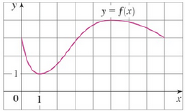Chapter 4.2, Problem 1E### Single Variable Calculus: Early Tr...

8th Edition
James Stewart
ISBN: 9781305270343

#### Solutions

Chapter
Section### Single Variable Calculus: Early Tr...

8th Edition
James Stewart
ISBN: 9781305270343
Textbook Problem

# The graph of a function f is shown. Verify that f satisfies the hypotheses of Rolle’s Theorem on the interval [0, 8]. Then estimate the value(s) of c that satisfy the conclusion of Rolle’s Theorem on that interval.To determine

To verify: Whether the function f(x) satisfies the conditions of Rolle’s Theorem on [0,8] ; estimate the value of c that satisfies the conclusion of Rolle’s Theorem on [0,8] .

Explanation

Rolle’s Theorem:

“Let a function f satisfies the following conditions,

1. A function f is continuous on the closed interval [a,b] .

2. A function f is differentiable on the open interval (a,b)

3. f(a)=f(b)

Then, there is a number c in an open interval (a,b) such that f(c)=0 .”

Verification:

The graph of the function f(x) is shown below in Figure 1.

From Figure 1, it is observed that the graph is continuous on the closed interval [a,b] as it has no breaks

### Still sussing out bartleby?

Check out a sample textbook solution.

See a sample solution

#### The Solution to Your Study Problems

Bartleby provides explanations to thousands of textbook problems written by our experts, many with advanced degrees!

Get Started

#### Simplify the expressions in Exercises 97106. (xy)1/3(yx)2/3

Finite Mathematics and Applied Calculus (MindTap Course List)

#### 272/3

Applied Calculus for the Managerial, Life, and Social Sciences: A Brief Approach

#### Think About It What is the x-coordinate of any point in the yz-plane?

Calculus: Early Transcendental Functions (MindTap Course List)

#### limx6(x210x)=. a) 24 b) 6 c) 26 d) does not exist

Study Guide for Stewart's Single Variable Calculus: Early Transcendentals, 8th

#### What is the goal of a hypothesis test?

Research Methods for the Behavioral Sciences (MindTap Course List)